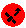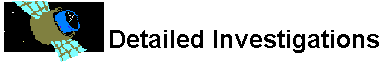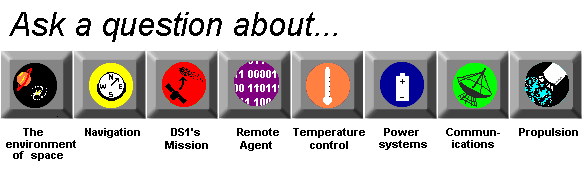# Space Environment

## Why do mass and distance affect gravity?

Gravity is a fundamental underlying force in the universe. The amount of gravity that something possesses is proportional to its mass and distance between it and another object. This relationship was first published by Sir Issac Newton. His law of universal gravitation says that the force (F) of gravitational attraction between two objects with Mass1 and Mass2 at distance D is:

F = G(mass1*mass2)/D squared.

(G is the gravitational constant, which has the same value throughout our universe.)

Einstein's theory of relativity adds to this. His theory predicted that objects with great mass deform space around them, causing light to deflect into them. That has been shown to be true. He also predicted that gravity could travel in gravity waves, which we haven't seen yet.

None of this explains why mass or distance affects gravity, though. To do that, we must look at the theories of scientists more recent than Einstein. According to theory, the reason mass is proportional to gravity is because everything with mass emits tiny particles called gravitons. These gravitons are responsible for gravitational attraction. The more mass, the more gravitons.

Graviton theory also accounts for differences in gravitational attraction over distances. Most gravitons exist in a cloud around the object. As distance from the object increases, the density of the graviton cloud goes down, so there is less gravitational attraction.What is gravity?Is there gravity in space?What is mass?Can gravity affect the surface of objects in orbit around each other?What is escape velocity?What role does the Sun play in space missions like DS1's?What's a gravity well?